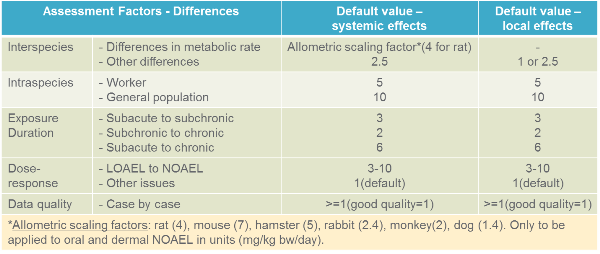CRA

## Introduction to Allometric Scaling and Its Use in Toxicology and Health Risk Assessment

Little Pro on 2019-10-20 Views:  Update:2019-11-16

Allometric scaling is a very important concept in toxicology and health risk assessment. It is commonly used for inter-species dose extrapolation and establishing a safe dose for humans based on animal toxicology data. In this article, we will give you a brief introduction to allometric scaling and how to use it in health risk assessment.

### What Is Allometric Scaling Important and What Is Allometric Scaling?

We often obtain no-observed-adverse-effect levels (NOAELs) or lowest-observed-adverse-effect levels (LOAELs) from studies in animals and use them to estimate safe dose levels for humans such as DNEL, ADI or RfD for humans by dividing NOAELs or LOAELs with overall assessment/uncertainty factors (UF). The default safety factor is 100 which takes into about of both interspecies difference (2.5 x 4) and intraspecies difference (3.16 x 3.16).In reality, we need to take into account of more factors such as realistic toxicodynamic and toxicokinetic differences (i.e, metabolic rate) between test animals and humans when choosing appropriate uncertainty factors. For example, it is probably not appropriate to apply the same uncertainty factors to NOAELs from rats and NOAELs from dogs or monkeys to extrapolate a safe dose level for human as dogs and monkeys are more similar to humans and smaller uncertainty factors can be justified. Sometimes we also need to estimate safe dose levels for 1 animal based on toxicology data from other animals. In this situation, we need to understand more realistic differences between different animals instead of applying the default uncertainty factors.

Allometric scaling is introduced to solve this issue. It refers to the scaling of physiological rates or quantities to relative growth and size (mass or volume) of one animal species relative to another animal species. It is based on agreed science that the effects of toxicological relevance are driven by the basal metabolic rate as it affects physiological processes such as cardiac output, blood flow and perfusion of liver and kidneys which, in turn, affect the elimination/clearance of most chemicals. Both European Commission and US EPA recommend the use of BW3/4 scaling. This relationship in toxicology can be written as:

Equitoxic dose <-> Physiological process = a x BW^(3/4), where:

a is a constant and BW refers to body weight.

### Examples of Allometric Scaling

Now let’s scale a single dose of 2.5mg in rat (body weight: 0.25kg) to humans (body weight=70kg) using allometric scaling principle. The scaled human Intake or oral dose will be:

Scaled human dose (in mg) = 2.5 x (70/0.25)^(3/4) = 170 mg

The scaled human dose is equivalent to 2.4 mg/kg bw (=170mg/70 kg). Please note that the unit is very important for scaling. We always use absolute intake or exposure (in mg, not in mg/kg bw) when doing BW3/4 scaling.

Let’s do another exercise for a dog (a single dose rate of 2.5mg, body weight: 20kg). The scaled human dose will be 9.25mg or 0.13mg/kg bw (9.25mg/70kg).

Scaled human dose (in mg) = 2.5 x (70/20)^(3/4) = 9.25 mg

Above relationship can be simply written as:

Scaled human dose (in mg) = Animal dose (in mg) x (BWh/BWa) ^(3/4)

We will use this shortly.

### Allometric Scaling Factor

In health risk assessment, it is more common to use body weight-based dose level (mg/kg body weight) to estimate safe dose levels. Let’s change previous equation a little bit by using a different expression for scaled human dose (in mg) and animal dose (in mg).

Scaled human dose (in mg/kg) * BWh = Animal dose (in mg/kg) * BWa x (BWh/BWa)^(3/4)

Then we can get the following equation:

Scaled human dose (in mg/kg) = Animal dose (in mg/kg) x (BWh/BWa)^(-1/4)

= Animal dose (in mg/kg) / [(BWh/BWa)^(1/4)]

Do you remember how we get estimated safe dose level for humans by diving animal dose levels (in mg/kg) with overall uncertainty factors at the beginning? Now you can see that 1 of the uncertainty factors is actually the allometric scaling factor and it can be written as:

Allometric Scaling Factor = (BWh/BWa)^(1/4)

### The Use of Allometric Scaling Factor in Risk Assessment

Allometric scalling factor is one of the uncertainty factors to consider addressing the differences between the experimental animal data and the human situation. European Chemicals Agency has calculated different allometric scaling factors for different species when compared to humans (see table below). The overall assessment factor is obtained by simple multiplication of individual assessment factors.Read more: How to calculate DNELs under REACH

Please note: Allometric scaling factors should not be applied in cases where doses in experimental animal studies are expressed as concentrations (e.g., in mg/m3 in air, ppm in diet, or mg/l in the drinking water) as these are assumed to be already scaled according to the allometric principle. However, once the concentration (e.g., ppm in diet) has been converted into a dose (e.g., mg/kg/day), an allometric scaling factor can be used. Allometric scaling does not apply to local effects either.

### References